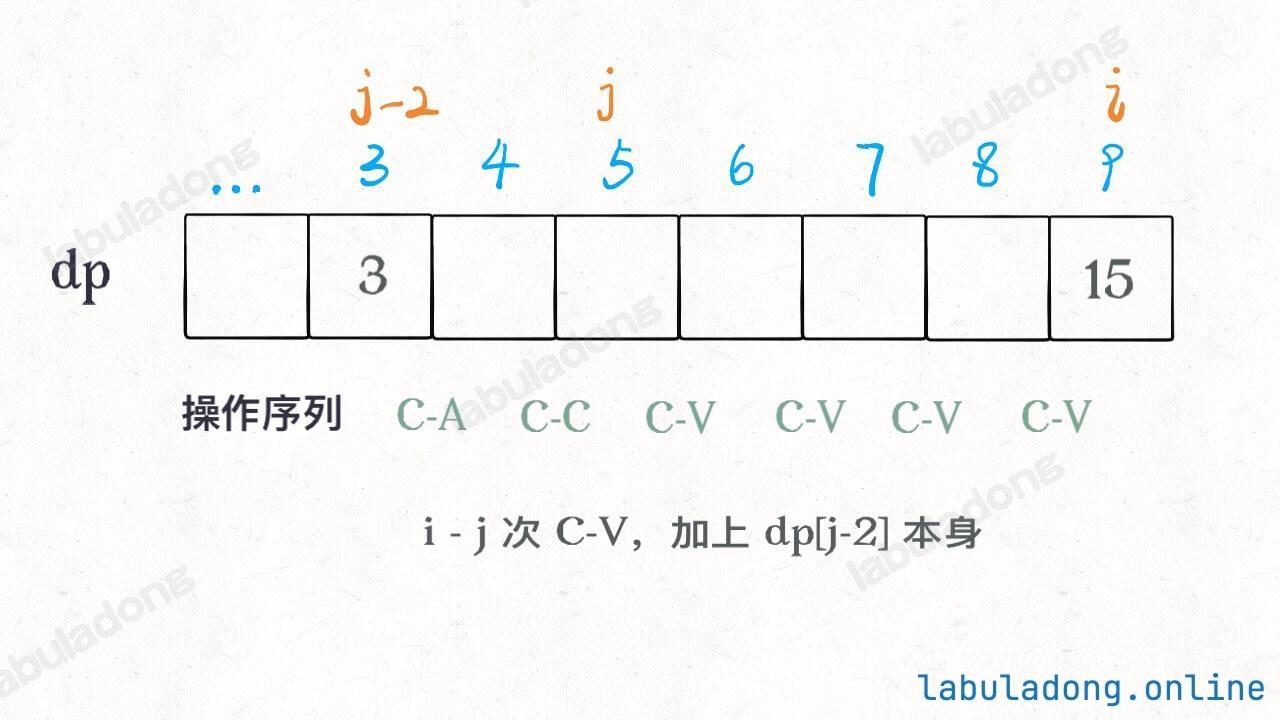Info

1、`A` 键：在屏幕上打印一个 `A`

2、`Ctrl-A` 键：选中整个屏幕。

3、`Ctrl-C` 键：复制选中的区域到缓冲区。

4、`Ctrl-V` 键：将缓冲区的内容输入到光标所在的屏幕上。

``````int maxA(int N);
``````

`A`, `A`, `A`, `Ctrl-A`, `Ctrl-C`, `Ctrl-V`, `Ctrl-V`

### # 第一种思路

``````dp(n - 1, a_num + 1, copy),    # A

dp(n - 1, a_num + copy, copy), # C-V

dp(n - 2, a_num, a_num)        # C-A C-C

``````

``````def maxA(N: int) -> int:

# 对于 (n, a_num, copy) 这个状态，
# 屏幕上能最终最多能有 dp(n, a_num, copy) 个 A
def dp(n, a_num, copy):
# base case
if n <= 0: return a_num;
# 几种选择全试一遍，选择最大的结果
return max(
dp(n - 1, a_num + 1, copy),    # A
dp(n - 1, a_num + copy, copy), # C-V
dp(n - 2, a_num, a_num)        # C-A C-C
)

# 可以按 N 次按键，屏幕和剪切板里都还没有 A
return dp(N, 0, 0)
``````

``````def maxA(N: int) -> int:
# 备忘录
memo = dict()
def dp(n, a_num, copy):
if n <= 0: return a_num;
# 避免计算重叠子问题
if (n, a_num, copy) in memo:
return memo[(n, a_num, copy)]

memo[(n, a_num, copy)] = max(
# 几种选择还是一样的
)
return memo[(n, a_num, copy)]

return dp(N, 0, 0)
``````

``````dp[n][a_num][copy]
# 状态的总数（时空复杂度）就是这个三维数组的体积
``````

### # 第二种思路

``````int[] dp = new int[N + 1];
// 定义：dp[i] 表示 i 次操作后最多能显示多少个 A
for (int i = 0; i <= N; i++)
dp[i] = max(
这次按 A 键，
这次按 C-V
)
``````

``````// 按 A 键，就比上次多一个 A 而已
dp[i] = dp[i - 1] + 1;
``````

``````public int maxA(int N) {
int[] dp = new int[N + 1];
dp = 0;
for (int i = 1; i <= N; i++) {
// 按 A 键
dp[i] = dp[i - 1] + 1;
for (int j = 2; j < i; j++) {
// 全选 & 复制 dp[j-2]，连续粘贴 i - j 次
// 屏幕上共 dp[j - 2] * (i - j + 1) 个 A
dp[i] = Math.max(dp[i], dp[j - 2] * (i - j + 1));
}
}
// N 次按键之后最多有几个 A？
return dp[N];
}
``````### # 最后总结

``````def dp(n, a_num, copy):
dp(n - 1, a_num + 1, copy),    # A
dp(n - 1, a_num + copy, copy), # C-V
dp(n - 2, a_num, a_num)        # C-A C-C
``````